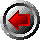Previous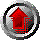Home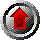Contents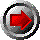Next

# Chapter 7: Types

This chapter describes the world of types in Aldor.

To use Aldor effectively, it is important to understand how to make type declarations. Type declarations allow one to write the same sorts of programs that one can write in C or Fortran. The base libraries provide a rich set of types and constructors, sufficient for many purposes. The chapter begins by describing how to create simple type expressions for declarations.

To take fuller advantage of Aldor, it is useful to understand how to create new types and environments. In this area, Aldor provides considerably more power than other programming languages. The remainder of the chapter explains the language primitives for forming new environments and shows how they may be used to provide parameterized types and packages.

### 7.1 : Why types?

Values are ultimately stored in a computer as sequences of bits. A given sequence of bits, however, can have different interpretations when used by different programs.

For example, on one computer the 32 bits

`01000101011010000011111100000000`

can represent

• the integer 1164459776,
• the floating point number 3.7159375 x 103, and
• the character string "Eh?".

A type provides an interpretation of binary data as a value which a program can manipulate. Different programming languages have different ways to associate types with data.

• Types on Data: Some languages, such as Lisp, incorporate types in values, augmenting the representation of a value with extra bits encoding the type. In such languages, each data value is self-identifying. The implementation of the bits which encode the type can be done either in hardware or software. One goal of optimizing compilers for these languages is to determine cases when it is possible to avoid storing and checking these type codes.

• Types on Variables: Other languages, such as Fortran, associate types with variables, either with declarations or by implicit rules. Associating a type with each variable requires less optimization to be efficient, but can be less flexible than the previous approach. Also, this method can provide a greater degree of safety, since certain mistakes can be detected before program execution.

• Types on Faith: Finally, some languages, such as B, a predecessor of C, have different operations to interpret data in different ways. Any data value can be used in any operation, and it is the responsibility of the programmer to get things right. For example, one operation will interpret a set of bits as pointer and another will interpret it as an integer.

Most modern programming languages associate types with values, with variables or both.

Object-oriented programming languages tend to adopt the `` Types on Data'' approach. Variables might be declared to belong to certain classes, but objects generally carry type information in the form of object-specific methods. Some object languages, such as C++, achieve efficiency by treating primitive and non-primitive types differently. The programmer must constantly remember the difference --- for example, it is not possible to derive new classes from int or char *. Other object languages uniformly pair types with data values, giving what is sometimes called ``objects all the way down.''

Aldor, on the other hand, adopts the `` Types on Variables'' approach, and values are not normally self-identifying. This approach allows uniform, efficient treatment of primitive and program-defined types. The flexibility that we have come to expect from object systems is obtained by promoting types to be first-class values.

Treating types as first-class values leads to a greater versatility than typical object systems. For example, programs may be parameterized by types provided at execution time. Many values may be declared as belonging to exactly the same execution-time type, or to types having some defined relationship. Combining operations can be made safe without requiring execution-time tests. The usual sort of object behaviour is easily recaptured. For example, self-identifying data may obtained by incorporating types in the values.

### 7.2 : Type expressions

Expressions in Aldor may compute values which are types. For example, all of the expressions in bold face in the program below produce type values:

```#include "aldor"
i :=  Integer;
l :=  List Integer;
af:  Array Float := [1.0, 2,0, 3.0];
myfun(T:  BasicType):  BasicType ==  Array T;
mytype :=  myfun Float;
```

In this example we see a number of expressions computing type values, some of which are assigned to variables, others passed as parameters, and still others used in declarations. Type values are typically formed by applying type-constructing functions to values of one sort or another.

Certain kinds of types in Aldor are used in specific ways.

A domain is a type which defines a collection of exported symbols. The symbols may denote types, constants and functions. Many domains also define an interpretation for data values, called a representation type; these domains are also known as abstract data types. Those domains which are not abstract datatypes are called packages.

A category is a type which specifies information about domains, including the specification of the public interface to a domain, which consists of a collection of declarations for those operations which may be used by clients of the domain.

The next several sections describe properties of types, which are common to all types. section 7.8 describes those properties of types which are specific to domains, packages and abstract datatypes. section 7.9 describes properties of categories.

The language defines a number of types and type-constructing functions. These are described in chapter 13. Most programs also use types or type constructors from various libraries. Section 14.2 describes standard libraries used with Aldor.

### 7.3 : Type context

Whereas any expression may be used to compute a type value, there are certain contexts in which a type is required. These contexts are indicated by T in the following expressions (details of which may be found by consulting the index):
 ... : T declaration ... : *T declaration ... \$ T selection ... :: T type conversion ... @ T type constraint ... pretend T type lie T with ... primitive type former T add ... primitive type former import ... from T bring names into scope inline... from T allow program dependency export ... from T export names from scope export ... to T exoprt names to foreign environment

We say that the expression T is in type context.

Type context is special in two ways:

• Expressions occurring in type context are not guaranteed to be evaluated in any particular order, or even evaluated at all.
• Identifiers occurring in an expression, T, appearing in type context must be constant in the scope in which T occurs.

Type evaluation

The reason expressions in type context are not guaranteed to be evaluated in order, or at all, is to give maximum flexibility to produce efficient programs. A portable program will only use non-side-effecting expressions in type context.

The expressions occurring in type context should be viewed as an annotation of a program rather than as part of the computation. For instance, the same program may be written with declarations, type restrictions and selections being inferred or explicit in varying degrees. So the program

```#include "aldor"
import from Integer;

n := 2 + 2;

print << n << newline
```

has the same meaning as

```#include "aldor"
import from Integer;

n: Integer := 2 +\$Integer 2;

print << n << newline
```

which has the same meaning as

```#include "aldor"
import from Integer;

I ==> Integer;

n: I := (+\$I @ (I,I) -> I) (2@I, 2@I);

print << n << newline
```

Name constancy

As a related but more fundamental concern, an expression in type context must only contain names which are constant over the scope in which the type occurs, because, without this rule, it would not be possible to associate well-defined types to expressions.

Consider the following incorrect program:

```#include "aldor"

f(n: Integer): () == {
local a, b: IntegerMod(n);

a := coerce((3 * n + 1) quo 2);
b := coerce((5 * n + 1) quo 3);

if n > 4 then   n := n + 1;  -- This line is ILLEGAL!

c := a - b;

print << "The result is " << c << newline
}
```

The problem is that if n is updated, the type of a and b is no longer valid and there is no reasonable interpretation for ``-'' or`` c''.

The names appearing in an expression in type context may be

• defined via ``=='',
• imported via ``import'', or
• function parameters which are nowhere updated.

### 7.4 : Dependent types

In many programming applications it is not possible to determine the specific type, of a family of related types, that an expression will have, until it is evaluated. There are a number of possible ways to treat this situation:

Solution 1: Forget the type information.

If it is known that the result is some kind of stored structure, then pretend it is a Pointer.

This solution has obvious drawbacks:

• The private representation information known about the result is compromised, creating a source-code dependency which can be difficult to manage.
• The result might not really be a pointer --- it might be an integer, a floating point number or something else which uses memory differently.
• Mistakes are more difficult to detect.

Solution 2: Use a Union type.

If there is a fixed set of possible result types, then one could return a Union type which includes the lot.

This solution is only acceptable when the set of types is fixed and the logic associated with each case is relatively distinct. If the different cases are treated in essentially the same way, then this solution encourages a programmer to treat the various union branches with the same code.

Solution 3: Use an Object-Oriented approach.

One can associate the dynamic type of the value with the value itself.

If the different possible types are to be handled in essentially the same way, then this solution can be a reasonable choice. The type information associated with the value can carry the functions which may be used to operate on it.

One difficulty with this solution is that it is not possible to indicate when many values belong to the same dynamic type, leading either to unsafe code, or to testing tags during program execution.

Solution 4: Use Dependent Types

A dependent type is a type T in which the type of one subexpression of T depends on the value of another.

Dependent types allow compile-time type checking for values whose types depend on other values which will only be present during program execution. This powerful tool is not often provided in other programming languages. In Aldor, it is a basic feature of the language which allows a great deal of flexibility in supporting various programming paradigms.

Dependent types

Consider the following example:

```#include "aldor"

sumlist(R: Ring, l: List R): R == {
s: R := 0;
for x in l repeat s := s + x;
s
}

import from List Integer, List SingleFloat;

print << sumlist(Integer,     [2,3,4,5]) << newline;
print << sumlist(SingleFloat, [2.0, 2.1, 2.2, 2.4]) << newline;
```

The type of the parameter ``l'' and the type of the result of the function each depend on the value of the parameter ``R''.

Because of this dependency, we say that the type of sumlist is a dependent type, in this case the dependent mapping type

```(R: Ring, l: List R) -> R
```

Note that within sumlist it was known that all the elements of the list l had type R. The ``+'' operation does not need to make any run-time checks to verify that both of its operands are of the same type.

Dependency is a relationship among values which arises because of the occurrence of one value in the type of another. In Aldor, dependencies between values are allowed in the following contexts:

• function parameters
• function results
• cross product values
• signatures in a category with-expression
In addition, the function result types are allowed to depend on the values of the function parameters.

Uses and examples
Dependent types allow parametric polymorphism, as in the sumlist function shown above. They also allow a program to compute a result, and return with the result a context for interpreting the result.

For example, a function could be declared of type:

```eigenValues: (R: Ring, Matrix R) ->
(E: AlgebraicExtension R, Vector E)
```

A function satisfying the above declaration could be defined to create a new arithmetic domain in which the eigenvalues of a matrix may be defined, and a vector of eigenvalues could then be created in that domain. Both the new domain and the vector of eigenvalues could then be returned by the function. Operations for manipulating the eigenvalues can then be retrieved from E to operate on values from the vector.

A complete example of a dependent type constructing function has been seen already in section 2.4. There the MiniList function takes a parameter S: BasicType and returns result of type LinearAggregate S.

An example manipulating dependent mapping values is given in section 23.9. An example treating dependent type-value pairs as objects is given in section 23.10.

With types as values in Aldor, dependent types allow the specification of relationships among types, which can be an extremely powerful programming tool.

Mutually dependent types

Values may have mutually dependent types. For example, the type constructing functions defined by the language include:

```->: (Tuple Type, Tuple Type) -> Type
Tuple: Type -> Type
```

As another example, functions may be declared with mutually dependent arguments:

```Ladder: (D: with {f: % -> E}, E: with {g: % -> D} ) -> Type
```

These examples are quite simple. For comparison, the Aldor interface to the AXIOM library includes one clique of 125 mutually dependent type constructors.

Note that these mutual dependencies are in the type dimension; this type of dependency is analogous to the more usual dependency between expressions in the value dimension:

```RecA == Record(head: A, tail: Union(nil: Pointer, b: RecB));
RecB == Record(head: B, tail: Union(nil: Pointer, a: RecA));
```

### 7.5 : Subtypes

Type declarations in Aldor associate properties with constants and variables. These declared names may then be used in expressions and the rules for well-formed expressions determine the properties associated with the built-up expressions.

The most important property of an expression is how to interpret the data representing the value of the expression. Every value in Aldor is a member of a unique domain which determines the interpretation of its data.

Sometimes it is necessary to associate additional properties with values, and to provide rules to manipulate the values on the basis of the properties they satisfy. Subtypes provide the mechanism for manipulating the additional properties associated with values.

A subtype is a type whose members lie in a particular domain and satisfy a particular property. The domain to which the members belong is the base domain of the subtype. The vocabulary of properties differs from one base domain to another.

A value in Aldor may be a member of any number of subtypes. By definition, the base domain for each of these subtypes is the same as the unique domain of the value.

We use Subtype(D) to denote the set of all subtypes on the base domain D. Suppose T1 and T2 are two subtypes from Subtype(D). If all the values belonging to T1 also belong to T2, then we say T1 is a subtype of T2 and write T1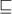T2. We also say that T2 is a supertype of T1 and write T2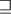T1. For any particular base domain D, the collection of subtypes Subtype(D) forms a lattice under the relation. The empty subtype is the bottom element and the subtype consisting of all elements of D is the top element. The subtypes based on one domain can have no relationship with subtypes based on another domain.

Not all domains support a vocabulary of properties. For this first version of the language, only the domain of all domains and the domain of all maps provide properties which lead to non-trivial subtype lattices. It would be a compatible extension to the language to allow arbitrary Boolean-valued functions to be used as properties for subtyping purposes.

The following rules define the relation:

• Categories: For any category-valued expression C, let Ex(C) denote the set of exported symbols which must be provided by any domain D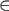C. Then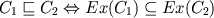As a corollary of the above definition: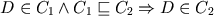Inheritance for domains from categories is analogous to class membership and inheritance between categories is analogous to class containment.

Note that Aldor is constructed so that a domain is only a member of a named category if it explicitly inherits from the category --- not if it merely exports the same collection of (explicit) declarations. For named categories C1 and C2, it is only the case that C1C2 if C1 inherits from C2, either directly or indirectly.

• Mapping types: The subtyping rule for mapping types is derived from the fact that a function which maps S1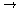T1 can be used in any context which provides a value of type S1 as an argument, or a value of type S2 where S2S1: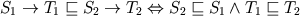Additionally, in determining the relation of mapping types, an argument or return with a keyword is a subtype of one without:

(A1, ..., ai: A1, ..., An)(A1, ..., Ai, ..., An)

If T1T2 then any value of type T1 can be used in any context which requires a value of type T2.

### 7.6 : Type conversion

A type conversion is an operation which changes a value from one type to a value of another type to which the original value would not otherwise belong. As a general rule, Aldor does not automatically convert a value from one type to another. For example, an integer is not automatically converted into a floating-point number. Such conversions must be made explicitly in the text of a program.

As discussed in section 7.5, a value in Aldor may be viewed as a member of more than one type by virtue of the subtype relation on types. In this case no conversion is necessary.

Primitive conversions

The language provides one primitive type conversion operation: pretend. A ``pretend'' expression is used to lie about the type of a value.

`Expr pretend Type`

causes the value computed by Expr to be treated as though it were of type Type. pretend is the only operator in Aldor which is not type-safe: using pretend can lead to unchecked type errors which only reveal themselves when a program is executed. For this reason pretend should be used with caution. For example, one could use ``pretend'' to examine the bit-level representation of data when a type does not provide operations to do so.

Two additional type-safe operations are defined in Aldor using pretend: rep and per. These operators convert between the public and private views of a type, and are discussed in section 7.8.

Conversion functions

Most type conversions are performed by functions. By convention, type conversion is most often performed by a ``coerce'' function, exported either by the source type or the destination type. Each library of Aldor programs may establish its own set of conventions regarding how conversion functions are named, and where they are implemented.

Examples of conversion functions could include:
 coerce: Integer -> % from DoubleFloat coerce: Integer -> % from Complex R coerce: DFlo\$Maching -> % from DoubleFloat coerce: % -> DFlo\$Machine from DoubleFloat coerce: R -> % from Complex R coerce: R -> % from Ratio R

Such functions are so common that a special syntax is defined to allow coerce functions to be called conveniently. The syntax

`Expr :: Type`

is a shorthand for the application

`coerce(Expr) @ Type`

(see section 8.3)

Courtesy conversions

While most type conversions must be made explicitly, a very conservative set of courtesy conversions are performed as needed. Courtesy conversions change between items represented as

Certain Aldor programs would be extremely pedantic if courtesy conversions were not applied.

The following courtesy conversions are applied automatically as required:

• Cross(T, ..., T) -> Tuple T
• Cross(T1, ..., TN) -> (T1, ..., TN)
• Cross(T) -> T
• (T, ..., T) -> Tuple T
• (T1, ..., TN) -> Cross(T1, ..., TN)
• T -> Tuple T
• T -> Cross T

These conversions allow functions which take or return multiple values to be used to pass arguments to other functions which can accept them, without requiring notation for an explicit conversion.

These conversions are applied only when the type of an expression exactly matches one of the conversions. For example, a value of type List Cross(T, T, T) would not automatically be converted to a value of type List Tuple T. Such a conversion could incur a significant hidden cost, even in more ordinary circumstances.

There is not at present any mechanism for a program to specify additional courtesy conversions.

### 7.7 : Type satisfaction

We say that a type S satisfies the type T if any value of type S can be used in any context which requires a value of type T.

The following rules define the satisfaction relation among types in Aldor:

• Exit: For any type T, the language-defined type Exit satisfies T. This rule allows an expression of type Exit to be used wherever any type is expected.

• Category: A type S satisfies the language-defined typeCategory if S is the type of a category.

• Type: A type S satisfies the language-defined type Type if S is the type of a domain or category. In other words, all domains and categories are types.

• No value: For any type S, the type S satisfies the type (). In other words, any value can be used in a context where no value is expected. The values are simply thrown away, and the expression is treated as having returned no value.

• Subtypes: If S is a subtype of T, then S satisfies T. See section7.5.

• Courtesy conversions: A type S satisfies a type T if a courtesy conversion exists which converts S to T. The courtesy conversion rules are described in section 7.6.

### 7.8 : Domains

A domain is an environment providing a collection of exported constants. These may include exported types, functions or values of other sorts.

It is useful to think of domains as being of two kinds: abstract datatypes and packages. An abstract datatype is a domain which defines a distinguished type and a collection of related exports. A package is a domain which does not define a distinguished type but does provide a collection of related exports. That is, a package is a domain which is not an abstract data type. This distinction is merely a convenience, though, as one is merely a special case of the other, as will be seen below.

Creating domains with ``add''

The primitive for forming domains is the add expression. The syntax for an add expression is

`[Expr] add AddBody`

The left-hand side Expr is an optional domain-valued expression, which specifies a domain from which any or all of the exports of the add can be inherited. Expr is called the parent (or parent domain) of the add.

The expression AddBody is typically a sequence of definitions which implement the exports of the domain.

Examples of the different kinds of domains, all of which can be created by add expressions, are to be found throughout the remainder of this section.

The value of the expression A add B is a domain which exports those symbols exported by A and those exports defined in B. The type of the expression A add B is

```C with { x1: T1; ...; xn: Tn }
```

here C is the type of A, and x1,...,xn are the symbols defined (using ==) in B, whose types are T1,...,Tn, respectively. Note that the types T1,...,Tn are allowed to contain references to the values x1,...,xn.

Packages

Packages are the simplest form of domains: they group a number of values together in an unordered collection that can be imported as a unit. A package formed with a single ``add'' expression can be used to provide functions operating in a common environment, and packages may be combined using binary ``add'' operations.

The following simple package provides functions for keeping score at a baseball game:

```add {
single(n: Integer): Integer == n;
double(n: Integer): Integer == n + n;
triple(n: Integer): Integer == n + n + n;
homer (n: Integer): Integer == n + n + n + n;
}
```

The exports from a package may include values belonging to different types, including various functions among different types. Conditional exports (see below) can also appear in packages.

Multi-sorted algebras

Since the exports of an add expression can include types and functions, packages can be used to implement multi-sorted algebras in a natural way: a multi-sorted algebra is just a package which exports two or more types. For example:

```#include "aldor"

NumberSorts == add {
Nat == Integer;
Rat == Ratio Nat;

rat(a: Nat, b : Nat): Rat == a / b;
num(r: Rat): Nat == numer r;
den(r: Rat): Nat == denom r;
}

import from NumberSorts;

n: Nat := 1 + 1 + 1;

print << r << newline;

r: Rat := rat(n - 1, n + 1);

print << r << newline;

s := rat(n - 1, n + 1);
print << s << newline;
```

This package exports the ``sorts'' (types) Nat and Rat, with operations for constructing values from each type.

Abstract datatypes

Often, a domain exports a distinguished type and a collection of operations on that type --- in general, enough operations to make the type a sufficiently interesting object. This situation is so overwhelmingly common that additional features in the language are used to support it.

An abstract datatype is a domain which defines a distinguished type and a collection of constants and functions related to the type.

The definition of an abstract datatype includes a specification of the representation of values belonging to the type and the implementation of operations which manipulate those values.

Inside an add expression which denotes an abstract datatype, the domain value created by the expression is used as the unique type exported by the expression. Within the add expression, this domain is denoted by the identifier ``%''.

We will often use the term ``domain'' when speaking of a particular abstract datatype, if the meaning is clear from the context. In abstract datatype terminology, a package may be described as a degenerate abstract datatype which does not provide any exports on its type. Such a structure is sometimes called an empty carrier.

The following example illustrates a simple abstract datatype:

```add {
I ==> Integer;
import from I;

0 : % == 0@I pretend %;
1 : % == 1@I pretend %;

(x: %) = (y: %) : Boolean == x@% pretend I = y@% pretend I;

~ (x: %) : % == if x = 1 then 0 else 1;
(x: %) \/ (y: %) : % == if x = 1 \/ y = 1 then 1 else 0;
(x: %) /\ (y: %) : % == if x = 1 /\ y = 1 then 1 else 0;
}
```

This domain expression includes constant definitions for identifiers ``0'' and ``1'' which belong to the domain and a few useful function definitions.

Since % denotes a domain, it can be used in type context just like any other type. In particular, operations defined in an add expression can be disambiguated by using a package call of the form ``x\$%'', which denotes a value named x defined in the add expression. Furthermore, inside B in a domain definition of the form:

```D : C == A add B
```

(described below, under ``Visibility of inherited operations'') the type expression D is treated as equivalent to the type expression %, since the domain D is defined as the value of the add expression, which is denoted by % inside B. The fact that % and D are equivalent is not visible outside the add. The equivalence is a property of the definition. The scope of % is bound by the add.

Outside the add expression, the type expression D has no relationship to any instances of % which may be visible.

Representation

The representation type of the elements of a domain is the domain which describes how data values belonging to the domain are encoded as sequences of bits.

Inside an add expression which denotes an abstract datatype, the identifier ``Rep'' is used to refer to the representation type of the elements of a domain. Since Rep is a domain, it can be used in type context just like any other type.

% and Rep denote two different types in Aldor. While a value of type % belongs to the public view of the domain, a value of type Rep belongs to the private view of the domain. For example, suppose that the representation type for a polynomial domain is a list domain. We would then say that a polynomial value is represented by a list: a list is not a polynomial, nor is a polynomial a list.

The operations ``rep'' and ``per'' provide a type-safe mechanism for converting data values between the public and private views of domain elements: rep converts a value of type % to the representation type Rep; per converts a value of type Rep to the public type %.

```rep x ==> x @ % pretend Rep;
per r ==> r @ Rep pretend %;
```

An easy way to remember which conversion operation to use is to remember the assertions rep(x)@Rep and per(r)@%: the result from rep has type Rep and the result from per has type % (percent).

To illustrate the use of Rep in a domain definition, consider the following version of the simple add expression given above:

```add {
Rep == Integer;
import from Rep;

0 : % == per 0;
1 : % == per 1;

(x: %) = (y: %) : Boolean == rep x = rep y;

~ (x: %) : % == if x = 1 then 0 else 1;
(x: %) \/ (y: %) : % == if x = 1 \/ y = 1 then 1 else 0;
(x: %) /\ (y: %) : % == if x = 1 /\ y = 1 then 1 else 0;
}
```

The representation type is defined as Integer, from which the domain imports the operations it needs to use. The constants 0 and 1 are defined by viewing corresponding values from the representation as values of the domain itself. Similarly, the equality operation is defined using the equality operation from the representation.

Separating a domain and its representation provides a clear line of demarcation between the public and private views of domain values. In this way, Aldor is an example of an abstract datatype language: the representation of a domain provides the private view of its elements which is used to define functions which operate on values from the domain. In public, values from the domain are viewed as belonging to the domain itself.

The operations provided by a domain can be arranged so that client programs which use a domain need not depend on the representation of the domain. When the representation of a domain is localized to the domain definition in this way, the representation is said to be encapsulated by the domain definition.

Each of the machine types is a domain whose representation depends on the architecture of the machine being used. While the actual representations of machine-level values are not specified by the language, typically most or all of them will have efficient runtime implementations. The availability of these built-in types provides a great degree of flexibility in designing efficient representations for Aldor domains.

Domain inheritance

A domain may inherit the implementation of many of its operations from another domain, called its parent, by placing the parent domain on the left-hand side of an add expression.

For example, when the add expression:

```Integer add {
Rep == Integer;
import from Rep;

0 : % == per 0;             -- This definition is redundant.
1 : % == per 1;             -- This definition is redundant.

-- This definition is redundant.
(x: %) = (y: %) : Boolean == rep x = rep y;

~ (x: %) : % == if x = 1 then 0 else 1;
(x: %) \/ (y: %) : % == if x = 1 \/ y = 1 then 1 else 0;
(x: %) /\ (y: %) : % == if x = 1 /\ y = 1 then 1 else 0;
}
```

is used to define a domain which exports the operations 0, 1, and =, these operations can be inherited from Integer, and so need not be implemented explicitly in the add expression.

A domain may inherit operations from another domain only if the representation type of the parent domain is compatible with the representation type of the domain. In many cases the representation type of the domain will be taken to be the parent domain itself. Packages and abstract data types may inherit from packages without reservation since there is no possibility of representation mismatch in this case.

Visibility of inherited operations

Operations provided by a domain are often defined using other operations provided by the domain. When some operations are inherited from a parent domain, it is important that these operations be visible in the add expression. In the domain definition

```MyBit : Logic with {
0 : %;
1 : %;
}
== Integer add {
~ (x: %) : % == if x = 1 then 0 else 1;
(x: %) \/ (y: %) : % == if x = 1 \/ y = 1 then 1 else 0;
(x: %) /\ (y: %) : % == if x = 1 /\ y = 1 then 1 else 0;
}
```

the definitions for ~, \/, and /\ each use the operations 0, 1, and = from MyBit, which are inherited from Integer. These inherited operations are made visible in the add expression by the following rule: whenever an expression A add B appears in a context requiring a domain whose type is the category C, then any operations required by C which are not defined in B are taken from the domain A.

So in the above example, the add expression used to define MyBit appears in a context which requires a domain which satisfies Logic and provides the exports 0 and 1. Logic is a category provided by the base Aldor library, which includes, among other things, a declaration for ``='':

```= : (%, %) -> Boolean;
```

Since the domain Integer (from the base Aldor library) also implements an operation = with the same signature, this operation is made visible on the right-hand side of the add.

Note that the statement import from Integer would also make the = operation from Integer visible, but its signature would instead be:

```= : (Integer, Integer) -> Boolean;
```

and so it could only be used to compare Integers, not MyBits.

Conditional definitions

A conditional definition is a definition which is only provided by a domain under certain assumptions. For example:

```Zmod (n: Integer) : Ring with {
if prime? n then Field;
}
== Integer add {
if prime? n then {
inv (x: %) : % == ...
}
}
```

Zn, the domain of integers modulo n, is always a Ring. However, if n? is prime, then Zn is also a Field, meaning that it should provide a multiplicative inverse for nonzero values. In an add expression, a definition which appears in the consequence of an if expression is said to be a conditional definition: the domain only provides the operation if the condition in the if expression evaluates as true.

### 7.9 : Categories

In Aldor a category is used to specify information about domains. Categories allow domain-manipulating programs to place restrictions on the sort of domains they are prepared to handle and to make promises about the domains which they may return. The restrictions and promises are expressed in terms of collections of exports which the domains in question will be required to provide.

All type values have ``Type'' as their unique base type. As with all other values, it is the unique base type which determines how values are to be represented.

On the other hand, a domain may belong to any number of categories so long as it has the necessary exports. That is, the world of categories provides a sub-typing structure on Type.

This section describes how to create and use categories.

Creating categories with ``with''

The primitive for forming categories is the with expression. The syntax for a with expression is

`[Expr] with WithBody`

The left-hand side is an optional Category-valued expression. Allowing a nonempty expression on the left-hand side is merely a syntactic convenience, and is equivalent to placing the expression as a part of the right-hand side:

`L with { R }`

is equivalent to

`with { L ; R }`

The right-hand side of a with expression contains a specification of the set of exports which must be provided for a domain to belong to this category. The specification is typically a sequence containing any of the following types of expression:

• declarations
• category-valued expressions
• conditional expressions
• default definitions

The following is an example of a simple category:

```with {
scale: (Integer, Integer) -> Integer;
n: Integer;
}
```

For a domain to satisfy this category, it must provide at least two exports: ``scale'', a function of the given type, and ``n'', an Integer. For example, the domain ``D'' as defined in the following program satisfies this category:

```D == add { import from Integer; n == 3; scale == *; extra == 0 }
```

Here, the type of D is inferred to be

```with {n: Integer; scale: (Integer, Integer) -> Integer; extra: Integer}
```

It is quite usual to see with expressions as the declared types in definitions. The definition of D could just as well have been written as

```D: with {
n:     Integer;
scale: (Integer, Integer) -> Integer;
extra: Integer
}
import from Integer;
n == 3;
scale == *;
extra == 0;
}
```

This form has the advantage that the interface to the domain is explicitly shown.

The exports specified by a with expression may have mutually dependent types. That is, it is possible to export types and operations on them. For example:

```with {
DecomposedMatrix: Type;

decompose: Matrix R -> DecomposedMatrix;

solve: (DecomposedMatrix, Vector R) -> Vector R
}
```

(where R is a parameter).

In general, any number of related types and operations may be given:

```#include "aldor"

with {
T: Type;
L: ListCategory T;

x: T
lx: L;
}
```

Typically, a domain will export a type and enough operations on that type to make it a sufficiently interesting object. It therefore makes sense to equate the exporting domain and the type for which the exports are being defined. The name used for this unified domain is ``%'', and is implicitly exported by all types formed using ``with'' and ``add''.

Thus, the ``DecomposedMatrix'' example above may be rewritten by replacing DecomposedMatrix with %:

```with {
decompose: Matrix R -> %;
solve: (%, Vector R) -> Vector R
}
```

A domain satisfying this type would have the following form:

```DecomposedMatrix: with {
decompose: Matrix R -> %;
solve: (%, Vector R) -> Vector R
} == add {
Rep ==> ...

decompose(m: Matrix R): % == ...
solve(dm: %, v: Vector R): Vector R == ...
}
```

Export from

In addition to the exports specified in the declarations of a with expression, a category may also cascade exports from other domains. That is, when a domain satisfying some category is imported, operations from other domains may be implicitly imported. The export from form is used to specify the additional domains to be imported.

For example,

```with {
decompose(m: Matrix R): % == ...
solve(dm: %, v: Vector R): Vector R == ...

export from Matrix R;
}
```

When a domain satisfying this category is imported, Matrix R will also be imported. As with the import from statement (see section 8.4), it is possible to restrict the imports which are cascaded:

```export {+: (%, %) -> %} from Matrix R;
```

This form will import only the ``+'' operation from Matrix R.

The cascaded exports of a category do not affect type satisfaction questions in any way.

Defaults

It is a common situation that, if we are given a category satisfying a number of operations, new operations can be defined on domains in that category, which only use the information supplied in the category. For example, a datatype with an equality operation may be declared as:

```with {
=:  (%, %) -> Boolean; ++ equality
}
```

A domain which satisfies this category is free to decide its own implementation of equality. As it stands, this is fine but we may want further operations on the datatype, such as a not-equals operation, ~=.

```with {
=:  (%, %) -> Boolean; ++ equality
~=: (%, %) -> Boolean; ++ inequality
}
```

This would imply that in order to satisfy this type a domain needs to export the two operations above. However, it seems a waste not to use the fact that inequality may be implemented in terms of equality in order to save every domain satisfying this category having to define both operations. This is achieved through default implementations (default constant bindings).

```with {
=:  (%, %) -> Boolean; ++ equality
~=: (%, %) -> Boolean; ++ inequality
default {
(a: %) ~= (b: %): Boolean == not (a = b);
}
}
```

The default implementation will call the ``='' operation from the domain, then return the complement.

A (rather trivial) domain satisfying this category is:

```Dom: with {
=:  (%, %) -> Boolean; ++ equality
~=: (%, %) -> Boolean; ++ inequality
default {
(a: %) ~= (b: %): Boolean == not (a = b);
}
} == add {
(a: %) = (b: %): Boolean == true;
}
```

This domain suffers from the deficiency that it is impossible to create new elements (due to the definition of equality), but importing from it will add the constants ``='' and ``~='' to the current scope.

A domain is also free to implement operations which have default implementations. In this case, the domain over-rides the operations in the default and importing the domain will activate the operations in the domain.

In a default definition, the uses of other exports from the type are obtained by looking up the operations in ``%''. This will first yield values from definitions in the domain or more closely applicable default bodies. Thus, default implementions provide a mechanism for late-binding of names to values.

Defining category-valued constants

It is inconvenient to have to repeat category expressions in a program. The language allows categories to be treated as normal values and allows names to refer to categories. A category (by definition) is a value of the Aldor built-in type Category.

To decide whether a particular domain satisfies a category, it is necessary to know that category's value. For this reason, it is most useful to use the ``define'' keyword in giving categories and category-returning functions their values. This makes the value as well as the type publicly visible.

```define Finite: Category == BasicType with {
#: Integer;
++ Number of values in the type.
...
}
```

defines a category, Finite, which exports a constant called ``#'' (size of a finite domain), plus some other things. ``BasicType here is a simple category which is near the root of the hierarchy of types of the Aldor base library. The following definition makes use of the Finite category:

```NotALot: Finite == add {
#: Integer == 0;
...
}
```

which creates a new domain ``NotALot'' for which the # constant has value 0. The remainder of the definition is elided here.

The new domain can then be used:

```import from NotALot;
print << #\$NotALot << newline;
```

The above program will print 0.

The new domain can also be used in contexts requiring something that satisfies Finite:

```-- define a function giving the size of a domain
sizeof(FiniteDom: Finite): Integer == #\$FiniteDom;
-- call it
sizeof(Boolean)       --- '2'
sizeof(SingleInteger) --- '2^n' for some n.
sizeof(NotALot)       --- '0' (as we said earlier)
```

The Finite category is actually part of the Aldor base library, and finite types such as Boolean and SingleInteger satisfy it.

A category can be used inside a ``with'' body. Including a category places all the declarations of that category into the new category. This mechanism allows categories to inherit from one another.

Thus, we can define a new category, ``FiniteGroup'' as:

```define FiniteGroup: Category == with {
Finite;
1:   %;             ++ Identity for multiplication.
*:   (%, %) -> %;   ++ Multiplication.
inv: % -> %;        ++ Inverses.
}
```

In order to satisfy this category, a domain must implement at least all the non-defaulted declarations from Finite, as well as the three explicitly mentioned by FiniteGroup.

Category defining functions

A ``with'' expression is a valid right hand side of a category definition. This allows the creation of parameterized categories. For example, the decomposed matrix category example above could be written as:

```define DecomposedMatrixCategory(R: Ring): Category == with {
decompose: Matrix R  -> %;
solve: (%, Vector R) -> Vector R
}
```

A domain which satisfies this category may be defined as follows:

```RatInt  ==> Ratio Integer;
DecomposedRationalMatrix: DecomposedMatrixCategory(RatInt) ==
Rep ==> ...

decompose(m: Matrix RatInt): % == ...
solve(dm: %, v: Vector RatInt): Vector RatInt == ...
}
```

A domain producing map can also satisfy this category. For example:

```Decomposed(R: Ring): DecomposedMatrixCategory(R) ==
Rep ==> ...

decompose(m: Matrix R): % == ...
solve(dm: %, v: Vector R): Vector R == ...
}
```

Join

The ``Join'' function takes as argument a tuple of categories and creates a new category which has the union of all their exports. Any conditions on declarations are ored together.

For example,

`Join(Order, Ring)`

produces the category:

`with { Order; Ring}`

which includes all the exports from both Order and Ring

Has expressions

A ``has'' expression has the following form:

`dom has cat`

where dom is a domain-valued expression, and cat is a category-valued expression. A ``has'' expression may be used in any part of a program, but is most often used to conditionalize domains and categories. The result of the expression is a Boolean value which will be true if dom can be shown to satisfy the category, and false otherwise.

Some examples:

```Integer has Ring                           -- true
Integer has Field                          -- false
Integer has with { +: % -> % }             -- true
Integer has with { +: Integer -> Integer } -- true

Integer has Ring with { next: % -> % }     -- true
Integer has Ring with { bozo: % -> % }     -- false

List Integer has Aggregate Integer         -- true
List Integer has Aggregate Float           -- false
```

The evaluation of this expression is made at run-time, so one may conditionalize code on the parameters to a function:

```sort!(T: Order, L: FiniteLinearAggregate T)(l: L): L == {
if L has ListCategory T then
listSort(L, l)
else
ordinarySort(L, l);
}
```

This function will either call ``listSort'' or ``ordinarySort'', depending on the type-valued parameter L.

```sort!(Integer, List Integer)    --- calls listSort
sort!(Integer, Array Integer)   --- calls ordinarySort
```

The category FiniteLinearAggregate(T) is satisfied by domains whose values are finite sized, and naturally indexable by integers. For example, lists, vectors and arrays but not trees, streams, integers and so on. An Order is a domain whose values may be ordered by a function ``<''.

Conditional expressions

Frequently, a domain will satisfy additional categories if particular conditions on parameters, or on the domain itself, are met. This information may be incorporated into a category expression as a conditional statement. A conditional statement has the same form as an if statement, except that if the body contains declarations and definitions, they are associated with the condition for the purposes of type satisfaction.

For example,

```with {
FiniteLinearAggregate S;
if S has Order then {
Order;
sort: % -> %;
}
}
```

is a category which is satisfied by any list type which exports an ordering on itself whenever its elements do. Provided that

```S == Integer;
```

the following domain would satisfy this condition:

```List Integer add {
Rep ==> List Integer;
(l1: %) < (l2: %): Boolean == {
local x, y: %;
for free y in tails l2 repeat {
x.first > y.first => return false;
x.first < y.first => return true;
}
if not empty? x then false;
}
sort(l: %): % == {
...
}
}
```

(``tails'' is a generator). Here, the condition is satisfied because S is Integer, an Order, and the appropriate operations are defined by the add body.

Conditional statements are most often used in parameterized categories:

```define ListCat(T: Type): Category == with {
if T has BasicType then BasicType;
FiniteLinearAggregate T;
...
}
```

Evaluation rules

The bodies of ``default'' statements inside with and add expressions may include side-effecting statements. These will be evaluated in order to make the constants inside the body well defined, but the language makes no guarantees on when (or indeed, if) these side-effecting statements will be evaluated for a given category or domain.

The bodies of with and add expressions are evaluated in such a way that they will be evaluated after the expressions they depend on. If mutually recursive type-forming expressions are found within the body of either with or add expressions, the expressions are computed as a fixed point, rather than evaluated in strict sequence. This fixed point computation uses a technique from functional programming to create self-referential data structures.PreviousHomeContentsNext